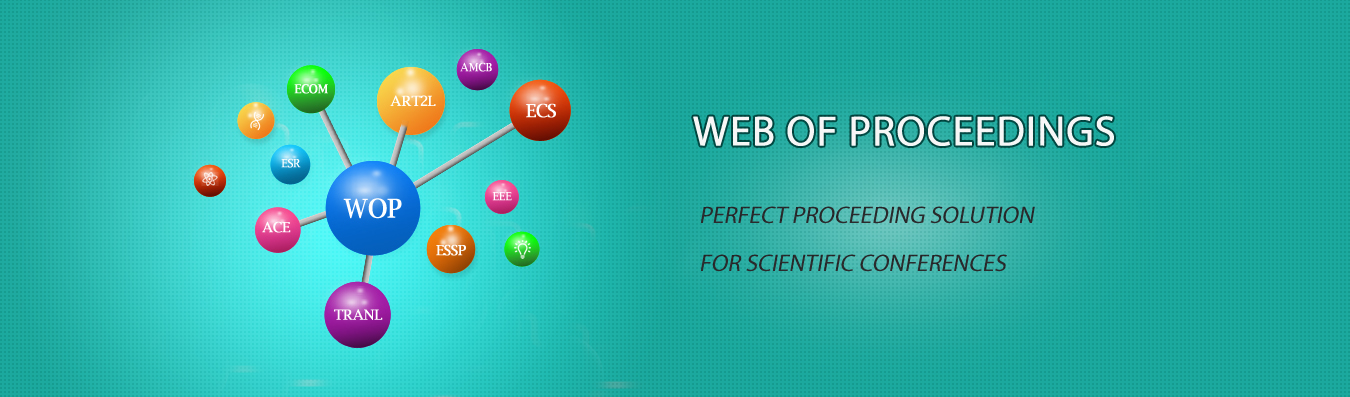The best way to conference proceedings by Francis Academic PressResearch on the Application of Mathematical Experimental Course System in the Teaching of Financial Mathematics Major in Colleges and Universities

DOI: 10.25236/icatpe.2019.273

Yinshan Jiang

Yinshan Jiang

Abstract

Students majoring in financial mathematics should not only have good financial analysis ability, but also learn to apply mathematical modeling methods to solve practical problems. Therefore, mathematics experiment teaching has become an indispensable part, and the premise of realizing effective mathematics experiment teaching is to establish a sophisticated experimental teaching course system. This paper analyzes the application of mathematics experimental course system in the teaching of financial mathematics in colleges and universities from four aspects: the status quo of financial mathematics and mathematics experiment, curriculum setting, design principles and curriculum optimization practice.

Keywords

Financial Mathematics; Mathematical Experiment Course; Curriculum Optimization